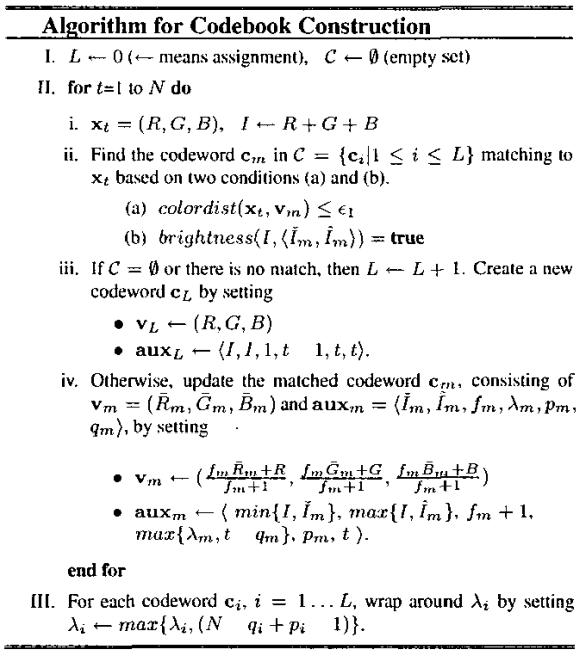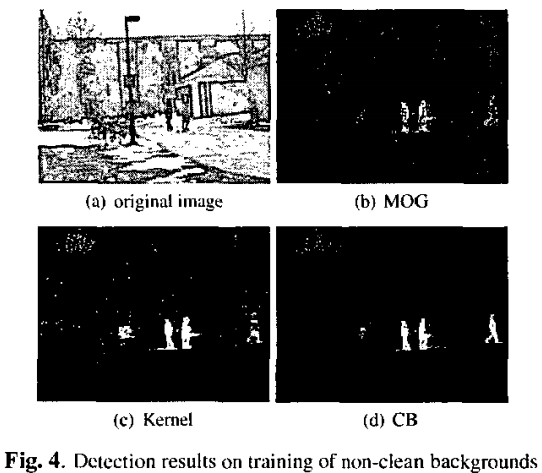Codebook is a typical clustering method, which can also be used for motion detection.

### Codeword structure

For each codeword in the codebook, the following information are stored: a. $$v$$: the average RGB color of the codeword. b. $$\hat{I}, \check{I}$$: the min and max brightness that the codeword accepted. c. $$f$$: the frequency with the codeword has occurred. d. $$\lambda$$: the maximum negative run-length defined as the longest interval during the training period that the codeword has not recurred. e. $$p, q$$: the first and the last access times that the codeword has occurred.

### Steps

1. For each pixel in the video, $$N$$ frames are first used to learn the codebook. In the training period, for each frame, the new pixel value is compared with the codebook. A codeword $$c_m$$ is matched if: i. the color distance between the new pixel value and the $$v_m$$ is no bigger than a threshold, and at the same time ii. the brightness of the new pixel is in the range of $$[\hat{I_m}, \check{I_m}]$$. a. If no codeword matches, a new codeword is created according to the new pixel value. b. If a codeword matches, all the parameters of the codeword (described in Codeword structure) are updated.

The codebook training algorithm is shown in Fig. 1.1. The background codebook is composed of those codewords leaned in Step 1 whose $$\lambda$$ are no bigger than $$N/2$$.

2. For the testing, when a new frame comes, the algorithm compares the new pixel with each codeword in the background codebook. If any codeword is matched, the new pixel is classified as background. Else, the new pixel is classified as foreground.

The results of codebook compared with GMM (or MOG) and KDE are shown in Fig. 2.